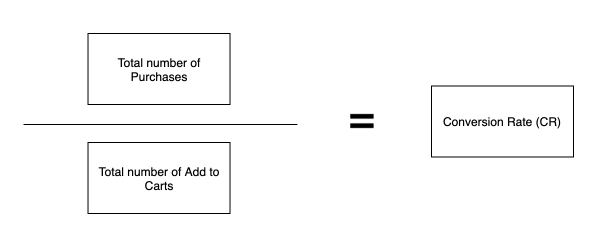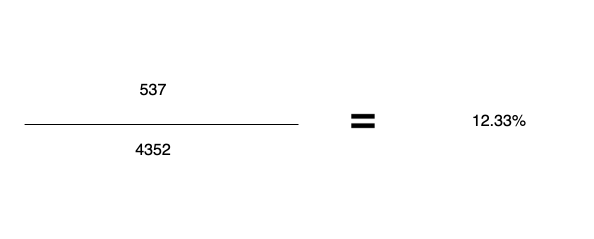Analytics
1. Help Center
2. Analytics

# How to calculate Conversion Rate for PickyStory?

## Calculate the conversion rate (Add to Cart to Purchase) to optimize revenue from the PickyStory app

Calculate the conversion rate (Add to Cart to Purchase) to optimize revenue from the PickyStory app. Follow the formula below to calculate the conversion rate for the PickyStory app:

Divide the total number of Purchases (from PickyStory only) by the total number of Add to Carts (from PickyStory only).

### General formula### Assumptions

• Total number of Add to Carts - 4352

• Total number of Purchases - 537Try PickyStory